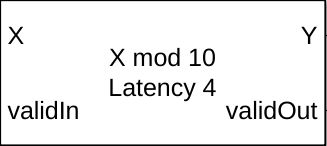# Modulo by Constant HDL Optimized

Perform mod operation with a constant denominator and generate optimized HDL code

• Library:
• Fixed-Point Designer HDL Support / Math Operations

•## Description

The Modulo by Constant HDL Optimized block performs the modulo operation (remainder after division) with a constant denominator using an HDL-optimized architecture with cycle-true latency.

The Modulo by Constant block uses an algorithm that is functionally similar to a Barrett Reduction. The division operation is computed via a multiplication by inverse, which generally results in better performance on embedded systems.

## Ports

### Input

expand all

Dividend, specified as a real scalar.

If X is a fixed-point data type, it must use binary-point scaling.

Data Types: `single` | `double` | `int8` | `int16` | `int32` | `int64` | `uint8` | `uint16` | `uint32` | `fixed point`

Whether input is valid, specified as a Boolean scalar. This control signal indicates when the data from the X input port is valid. When this value is `1` (`true`), the block captures the value on the X input port. When this value is `0` (`false`), the block ignores the input samples.

Data Types: `Boolean`

### Output

expand all

Result of modulus operation, returned as a scalar.

Data Types: `single` | `double` | `int8` | `int16` | `int32` | `int64` | `uint8` | `uint16` | `uint32` | `fixed point`

Whether the output data is valid, returned as a Boolean scalar. When the value of this control signal is `1` (`true`), the block has successfully computed the output Y. When this value is `0` (`false`), the output data is not valid.

Data Types: `Boolean`

## Parameters

expand all

Divisor to use for the modulus operation, specified as a positive, real-valued, finite scalar.

#### Programmatic Use

 Block Parameter: `Denominator` Type: character vector Values: MATLAB® expression that evaluates to a positive, real-valued, finite fixed point or numeric value Default: `'10'`

Data Types: `single` | `double` | `int8` | `int16` | `int32` | `int64` | `uint8` | `uint16` | `uint32` | `uint64` | `Boolean` | `fixed point`

## Extended Capabilities